# Binding Energy and Spin-Orbit Splitting of a Hydrogenic Donor Impurity in AlGaN/GaN Triangle-Shaped Potential Quantum Well

An Erratum to this article was published on 17 September 2009

## Abstract

In the framework of effective-mass envelope function theory, including the effect of Rashba spin-orbit coupling, the binding energyE b and spin-orbit split energy Г of the ground state of a hydrogenic donor impurity in AlGaN/GaN triangle-shaped potential heterointerface are calculated. We find that with the electric field of the heterojunction increasing, (1) the effective width of quantum well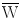decreases and (2) the binding energy increases monotonously, and in the mean time, (3) the spin-orbit split energy Г decreases drastically. (4) The maximum of Г is 1.22 meV when the electric field of heterointerface is 1 MV/cm.

## Introduction

GaN, AlN and their related compounds, as wide gap semiconductors, attracted a lot of interest in the past few yeas, mainly due to their good optical properties and great potential uses in optoelectronics. Spin-orbit coupling is the key issue of semiconductor spintronics . Since there is a large spin-splitting of a 2-dimensional electron gas (2DEG) in a wurtzite AlGaN/GaN heterostructrure , GaN-based materials as a promising candidate for the spintronic application have drawn a considerable attention. The spin-orbit interaction contains the Rashba and Dresselhaus contributions . As commonly known, the Rashba effect is due to the structural inversion asymmetry of confinement potential at heterointerface and the Dresselhaus effect is due to the bulk inversion asymmetry of crystal potential. Although spin-orbit coupling in wide band gap materials is thought to be weak , the surprisingly sizable spin splitting of the conduction band in GaN-based heterostructures mainly results from a large polarization doping effect and a strong interfacial electric field directed along the growth axis induced by piezoelectric effect . Strong polarization doping effect in GaN, AlN and their related compounds may compensate to some extent for the smallness of the coupling parameter and make overall spin-splitting comparable to that found in narrow-gap group-III-V structures. This fact could make GaN, AlN and their related compounds competitive in emerging spintronics applications.

Impurity states have played an important role in the semiconductor devices. Only with impurities, the devices, such as diodes, transistors can be successfully made . The study of impurity states in heterostructures is an important aspect to which many theoretical and experimental works have been devoted. Since Bastard’s pioneering work using a variational approach [11, 12], a lot of attention has been devoted to the study of impurity states in quantum wells . Previously, lots of researches focused on the GaAs/GaAlAs structures. Mailhiot, Chang, and McGill [14, 15] have done extensive calculations of the binding energies and wave functions for hydrogenic impurities as functions of well width and impurity location in the well using a more realistic model in which the potential barrier was finite and equal to the conduction band offset at GaAs/GaAlAs interfaces. Chaudhuri  has extended this to treat the case where the GaAlAs layer is thin enough that the wave functions can spill over to adjacent GaAs quantum wells. Recently impurities in GaN based structures began to draw a lot of attentions. Xia and his coworkers  calculated the binding energy of a hydrogenic impurity in zinc-blende (ZB) GaN/AlN coupled quantum dots (QDs) using a variational method. Both of them found that: (1) the binding energy increases when the width of quantum well decreases; (2) the impurity’s binding energy depends upon its location within the well. The peak occurs when the impurity is on the midpoint of the quantum well.

## Calculations

In the framework of effective-mass envelope function theory , we studied the binding energy of the hydrogenic impurity state first, and then the electronic states of a hydrogenic donor impurity in AlGaN/GaN triangle-like potential heterojunction including the effect of Rashba spin-orbit coupling will be calculated in this letter.

The electron envelope function equation with a hydrogenic donor impurity located atr0 = (0,0,z0) is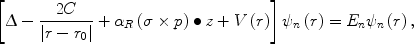(1)

where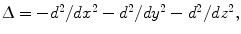r = (x,y,z), and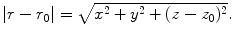. The third item in Eq. (1) is the contribution of the Rashba spin-orbit effect to the single electron Hamiltonian.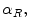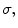and p are the Rashba parameter, the Pauli matrices, and the electron momentum operator, respectively. The units of length and energy are given in terms of the effective Bohr radius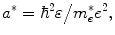and the effective Rydberg constant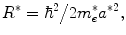where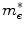and ɛ are the electron effective mass and dielectric constant of an electron in GaN, respectively.

In Eq. (1),C = 0 for the case of no donor; and 1 for the case if there is a donor in the heterointerface. The binding energy of the hydrogenic impurity state is calculated by the following equation: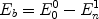(2)

To a good approximation, the infinite triangle-shaped confined potential well was used to calculate the conduction band ground state. Such potential is given by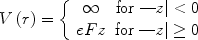(3)

whereF is the electric field, which depends on the Al componentx of the barrier layer Al x Ga1−xN and 2DEG density. To compare with square quantum well, effective width will be used. Effective width of triangle-shaped well is usually given by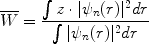(4)

where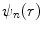is the eigen wave function ofE n solved from the Schrodinger equation with the triangle-shaped confined potential well and so different staten has different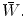For ground state,n = 0, furthermore, Eq. (4) can be transformed to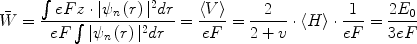(5)

whereF is the electric field and the coefficientν of the linear potential is unity.

In this calculation, the normalized plane-wave expansion method is used. We employ the electron wave function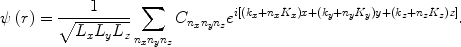(6)

where L x , L y , and L z are the side lengths of the unit cell in the x y, and z directions of the coordinate system, respectively. K x = 2π/L x , K y = 2π/L y , K z = 2π/L z , n x {−m x ,…,m x }, n y {−m y ,…,m y }, n z {−m z ,…,m z }. The plane wave number is N xyz = (2m x + 1)(2m y + 1)(2m z + 1), where m x m y m z are positive integers. In our calculation, we adopt m x = m y = m z = 6. If further increasing integers m x m y m z , the difference between exact results and real one is negligibly small. During our calculation, we make k x = k y = k z = 0 in Eq. (6) . The electron states are calculated from the matrix elements which can be found in Eqs. (1) and (5) . For GaN, we take effective mass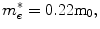with m0 being the free electron mass. The Rashba parameter is chosen to be 20 meV Å .

## Results and Discussion

Figure 1shows the effective well width of the triangle-like quantum well as a function of electric field for ground state. In the general case, theis proportional to about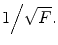The higher the electric field is, the narrower the effective well width will be. In our case, whenF = 100 MV/cm, it corresponds to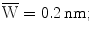while, forF = 1 MV/cm, it corresponds to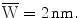In the limiting case,will tend to infinity when theF approaches to 0.

Figure 2 shows the binding energy of the ground state as a function of effective width of quantum well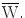In this figure, we assume that the impurity position is located at z0 = 0. From this figure, we can see two facts: (1) the binding energy splits into two-ones for the Rashba effect, but such split is quite small so that it can not be showed clearly in Figure 2; (2) when the effective well width is less than 0.5 nm, the binding energy increases dramatically and tends to infinite when theapproaches to 0. E b = 138 meV for the case of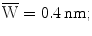and (3) When the effective well width is more than 1.0 nm, the binding energy tends to be constant about 28 meV. The reason is that when the effective well width of the triangle quantum well becomes narrower and the quantum confinement becomes stronger, so the binding energy increases as well, this tendency, as commonly known, is qualitatively similar to the case of square quantum well (e.g., AlGaAs/GaAs quantum well) .

Figure 3shows the spin-orbit split energy of ground state, labeled as Г, as a function of electric field F. The inset also shows the Г as a function of effective width of the quantum well as the impurity position pinned atz0 = 0. From this figure, we can also observe that (1) with the electric field increasing, thedecreases, which leads to a decreasing spin-orbit split energy Г, and (2) whenF is weaker than 2.5 MV/cm, Г will saturate at about 0.75 meV.

Figure 4 shows the variations in spin-orbit split energy of ground state Г as a function of impurity position in the different electronic fields. In this figure, we show the Г against typical electric field values of F = 1 MV/cm, 5 MV/cm, and 10 MV/cm, respectively, for a comparison. It was found that (1) in each field of F, with z0 located in a range from 0 to 10 nm, the spin-orbit splitting energy Г increases first, and then reaches its maximum value before decreasing monotonously, and we also found that our calculated curve is in agreement with Bastard’s calculation qualitatively . However, based on the Bastard’s work, we further calculated the ground state spin-orbit splitting energy for his ground state, and found that splitting energy shows a symmetrically spatial distribution, which is very different from that in our triangle-shaped well in curve shapes. To our knowledge, up to now, there has been no report on triangle-shaped quantum well binding energy calculation and triangle-shaped well spin-orbit splitting energy. Another worthy phenomenon from the same figure is that (2) When F increases, the maximum of spin-orbit splitting energy decreases drastically, because such spin splitting energy is very sensitive to the intrinsic and extrinsic electric field perpendicular to the quantum well plane and (3) with the increasing of electric field, the maximum of the spin splitting energies shows a systematically left shift. This is thought to be due to the strong infinite confined potential. Such strong potential can result in a narrower electron effective well width, in which no confined electron can escape from the well. When we chose the lowest electric field F = 1 MV/cm, we found a maximum Г of 1.22 meV at z0 = 2.3 nm, indicating that the weaker the electric field is, the bigger the spin-orbit splitting energy will be.

As commonly known, for wide band gap semiconductors, for instance, in our GaN and its related compounds, the spin-orbit splitting energies are much smaller than the quantum well subband and also the impurity binding energy. However, for the narrow band gap semiconductors, e.g., InSb, GaSb, AsIn or InN, the spin splitting effect cannot be negligible. Though in this paper we have studied spin-orbit effect in a wide band gap material, our work could hint other researchers who engage in spin-orbit effect in narrow band gap materials.

## Summary

In summary, we calculate the binding energy and spin-orbit split energy Г of the ground states of a hydrogenic donor impurity in AlGaN/GaN heterojunction in the framework of effective-mass envelope function theory, including the effect of Rashba spin-orbit coupling. When taking into account the GaN/AlGaN intrinsic polarization field and extrinsic applied electric field, We find that when such electric fields of the heterojunction increases, it can result in a decrease in effective well width of the triangle-shaped quantum well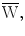and an increase of the binding energy. In the mean time, the spin-orbit split energy Г decreases drastically. In addition, we also find that the maximum value of Г could be up to 1.22 meV when the lowest electric field of 1 MV/cm crosses the AlGaN/GaN heterointerface.

## Misc

An erratum to this article can be found athttp://dx.doi.org/10.1007/s11671-009-9437-0

## References

1. Wolf SA: J. Supercond.. 2000, 13: 195. COI number [1:CAS:528:DC%2BD3cXitVahtbk%3D] COI number [1:CAS:528:DC%2BD3cXitVahtbk%3D] 10.1088/0953-2048/13/2/314

2. Žutić I, Fabian J, Sarma SD: Rev. Mod. Phys.. 2004, 76: 323. Bibcode number [2004RvMP...76..323Z] Bibcode number [2004RvMP...76..323Z] 10.1103/RevModPhys.76.323

3. Fabian J, Matos-Abiague A, Ertler C, Stano P, Žutić I: Acta Phy. Slovaca. 2007, 57: 565. COI number [1:CAS:528:DC%2BD2sXhtlCns7jN] COI number [1:CAS:528:DC%2BD2sXhtlCns7jN]

4. Litvinov VI: Appl. Phys. Lett.. 2006, 89: 222108. Bibcode number [2006ApPhL..89v2108L] Bibcode number [2006ApPhL..89v2108L] 10.1063/1.2397559

5. Kurdak C, Biyikli N, Özgür Ü, Morkoc H, Litvinov VI: Phys. Rev. B. 2006, 74: 113308. Bibcode number [2006PhRvB..74k3308K] Bibcode number [2006PhRvB..74k3308K] 10.1103/PhysRevB.74.113308

6. Winkler R: Spin-Orbit Coupling Effects in Two-Dimensional Electron and Hole Systems, Springer Tracts in Modern Physics. Springer, Berlin; 2003.

7. Ridley BK: Appl. Phys. Lett.. 2000, 77: 990. COI number [1:CAS:528:DC%2BD3cXls1Wmtbw%3D]; Bibcode number [2000ApPhL..77..990R] COI number [1:CAS:528:DC%2BD3cXls1Wmtbw%3D]; Bibcode number [2000ApPhL..77..990R] 10.1063/1.1288817

8. Litvinov VI: Phys. Rev. B. 2003, 68: 155314. Bibcode number [2003PhRvB..68o5314L] Bibcode number [2003PhRvB..68o5314L] 10.1103/PhysRevB.68.155314

9. Schmult S, Manfra MJ, Punnoose A, Sergent AM, Baldwin KW, Molnar RJ: Phys. Rev. B. 2006, 74: 033302. Bibcode number [2006PhRvB..74c3302S] Bibcode number [2006PhRvB..74c3302S] 10.1103/PhysRevB.74.033302

10. Yoffe AD: Adv. Phys.. 2001, 51: 1. Bibcode number [2001AdPhy..50....1Y] Bibcode number [2001AdPhy..50....1Y] 10.1080/00018730010006608

11. Bastard G: Phys. Rev. B. 1981, 24: 4714. COI number [1:CAS:528:DyaL3MXmt1Grtbg%3D]; Bibcode number [1981PhRvB..24.4714B] COI number [1:CAS:528:DyaL3MXmt1Grtbg%3D]; Bibcode number [1981PhRvB..24.4714B] 10.1103/PhysRevB.24.4714

12. Bastard G: Surf. Sci.. 1982, 113: 165. COI number [1:CAS:528:DyaL38XhtlSjsrw%3D]; Bibcode number [1982SurSc.113..165B] COI number [1:CAS:528:DyaL38XhtlSjsrw%3D]; Bibcode number [1982SurSc.113..165B] 10.1016/0039-6028(82)90580-5

13. Porras-Montenegro N, Pérez-Merchancano ST: Phys. Rev. B. 1992, 46: 15. and references therein and references therein 10.1103/PhysRevB.46.9780

14. Maihiot C, Chang Y, McGill TC: Phys. Rev. B. 1982, 26: 4449. Bibcode number [1982PhRvB..26.4449M] Bibcode number [1982PhRvB..26.4449M] 10.1103/PhysRevB.26.4449

15. Maihiot C, Chang Y, McGill TC: J. Vac. Sci. Technol.. 1982, 21: 519. Bibcode number [1982JVST...21..519M] Bibcode number [1982JVST...21..519M] 10.1116/1.571751

16. Chauduri S: Phys. Rev. B. 1983, 28: 4480. Bibcode number [1983PhRvB..28.4480C] Bibcode number [1983PhRvB..28.4480C] 10.1103/PhysRevB.28.4480

17. Xia CX, Liu YM, Wei SY: Phys. Lett. A. 2008, 372: 6420. COI number [1:CAS:528:DC%2BD1cXhtFOgtr7O]; Bibcode number [2008PhLA..372.6420X] COI number [1:CAS:528:DC%2BD1cXhtFOgtr7O]; Bibcode number [2008PhLA..372.6420X] 10.1016/j.physleta.2008.08.062

18. Greene RL, Bajaj KK: Solid State Commun.. 1983, 45: 825. COI number [1:CAS:528:DyaL3sXhtlGqsb4%3D]; Bibcode number [1983SSCom..45..825G] COI number [1:CAS:528:DyaL3sXhtlGqsb4%3D]; Bibcode number [1983SSCom..45..825G] 10.1016/0038-1098(83)90809-8

19. Greene RL, Bajaj KK: Phys. Rev. B. 1985, 31: 913. COI number [1:CAS:528:DyaL2MXosVKhtw%3D%3D]; Bibcode number [1985PhRvB..31..913G] COI number [1:CAS:528:DyaL2MXosVKhtw%3D%3D]; Bibcode number [1985PhRvB..31..913G] 10.1103/PhysRevB.31.913

20. Li SS, Xia JB: Appl. Phys. Lett.. 2008, 92: 022102. Bibcode number [2008ApPhL..92b2102L] Bibcode number [2008ApPhL..92b2102L] 10.1063/1.2833692

21. Li SS, Xia JB: Phys. Lett. A. 2007, 366: 120. COI number [1:CAS:528:DC%2BD2sXkvFGntLw%3D]; Bibcode number [2007PhLA..366..120L] COI number [1:CAS:528:DC%2BD2sXkvFGntLw%3D]; Bibcode number [2007PhLA..366..120L] 10.1016/j.physleta.2007.02.028

22. Li SS, Chang K, Xia JB: Phys. Rev. B. 2005, 71: 155301. Bibcode number [2005PhRvB..71o5301L] Bibcode number [2005PhRvB..71o5301L] 10.1103/PhysRevB.71.155301

23. Belyaev AE, Raicheva VG, Kurakin AM, Klein N, Vitusevich SA: Phys. Rev. B. 2008, 77: 035311. Bibcode number [2008PhRvB..77c5311B] Bibcode number [2008PhRvB..77c5311B] 10.1103/PhysRevB.77.035311

## Acknowledgments

This work was supported by the 863 High Technology R&D Program of China (Grant Nos. 2007AA03Z402 and 2007AA03Z451), the Special Funds for Major State Basic Research Project (973 program) of China (Grant No. 2006CB604907), and the National Science Foundation of China (Grant Nos. 60506002 and 60776015).

## Author information

Authors

### Corresponding author

Correspondence to Jun Wang.

## Rights and permissions

Open Access This article is distributed under the terms of the Creative Commons Attribution 2.0 International License ( https://creativecommons.org/licenses/by/2.0 ), which permits unrestricted use, distribution, and reproduction in any medium, provided the original work is properly cited.

Reprints and Permissions

Wang, J., Li, SS., Lü, YW. et al. Binding Energy and Spin-Orbit Splitting of a Hydrogenic Donor Impurity in AlGaN/GaN Triangle-Shaped Potential Quantum Well. Nanoscale Res Lett 4, 1315 (2009). https://doi.org/10.1007/s11671-009-9398-3

• Accepted:

• Published:

• DOI: https://doi.org/10.1007/s11671-009-9398-3

### Keywords

• Binding energy
• Spin-orbit splitting
• Hydrogenic donor impurity
• AlGaN/GaN